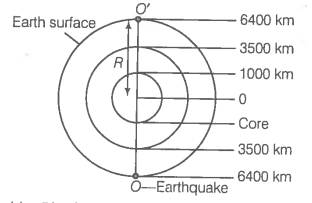The earth has a radius of 6400 km. The inner core of 1000 km radius is solid. Outside it, there is a region from 1000 km to a radius of 3500 km which is in a molten state. Then again from 3500 km to 6400 km the earth is solid. Only longitudinal (P) waves can travel inside a liquid.
Assume that the P wave has a speed of 8 km s-1 in solid parts and of 5 km s-1 in liquid parts of the earth. An earthquake occurs at some place close to the surface of the earth. Calculate the time after which it will be recorded in a seismometer at a diametrically opposite point on the earth if wave travels along diameter?

Hint: The speed is defined as the distance travelled per unit time.
Step 1: Use the formula of speed.
Speed of wave in solid= 8 km/s.Speed of wave in liquid= 5km/s
Required time=
=
=[125+500+362.5]$×$2 =1975
As we are considering at diametrically opposite point, hence there is a multiplication of 2.